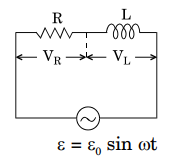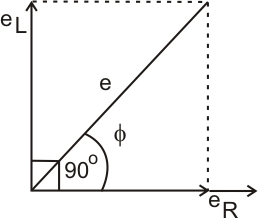# (a) An ac circuit as shown in the figure has an inductor of inductance L and a resistor of resistance R connected in series. Using the phasor diagram, explain why the voltage in the circuit will lead the current in phase.(b) The potential difference across the resistor is 160 V and that across the inductor is 120 V. Find the effective value of the applied voltage. If the effective current in the circuit be 1·0 A, calculate the total impedance of the circuit.(c) What will be the potential difference in the circuit when direct current is passed through the circuit?(a) In an inductor voltage leads current byand in resistor both voltage and current are in the same phase, the resultant ofANDthat isin the diagram will lead by phaseSo the resultant current will also lead by a phase(b) Given;-Now, calculating the impedance (Z) of the circuit.(c) When direct current is passed through the circuit the potential difference across the inductor is zero.

The potential difference of the circuit = potential difference across the resistor

### Preparation Products

##### Knockout NEET May 2021 (One Month)

An exhaustive E-learning program for the complete preparation of NEET..

₹ 14000/- ₹ 6999/-
##### Foundation 2021 Class 10th Maths

Master Maths with "Foundation course for class 10th" -AI Enabled Personalized Coaching -200+ Video lectures -Chapter-wise tests.

₹ 350/- ₹ 112/-
##### Foundation 2021 Class 9th Maths

Master Maths with "Foundation course for class 9th" -AI Enabled Personalized Coaching -200+ Video lectures -Chapter-wise tests.

₹ 350/- ₹ 112/-
##### Knockout JEE Main April 2021 (One Month)

An exhaustive E-learning program for the complete preparation of JEE Main..

₹ 14000/- ₹ 6999/-